# What is Full Wave Rectifier?

Power diodes can be connected together to form a full-wave rectifier that converts an AC voltage to a pulsating DC voltage for use in the power supply. We know that connecting a smoothing capacitor to the load resistor can reduce voltage ripple or DC voltage fluctuations. This method is suitable for low power applications, but not for applications that require a "stable and smooth" DC supply voltage. One way to develop this is to use it every half cycle of the input voltage, not every half cycle.

The circuit that makes this possible is called a full-wave rectifier. Like half-wave circuits, full-wave rectifier circuits produce an output voltage or current that has a constant or constant component. Full wave rectifiers have some fundamental advantages over their half wave rectifier counterparts. The average (DC) output voltage is higher than for half wave, the output of the full wave rectifier has much less ripple than that of the half wave rectifier producing a smoother output waveform.

In a Full Wave Rectifier circuit two diodes are now used, one for each half of the cycle. A multiple winding transformer is used whose secondary winding is split equally into two halves with a common center tapped connection, (C).  This configuration results in each diode conducting in turn when its anode terminal is positive with respect to the transformer center point C producing an output during both half cycles, twice that for the half wave rectifier so it is 100% efficient as shown below.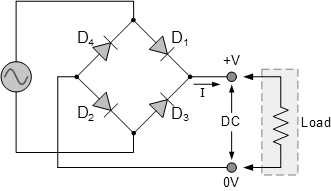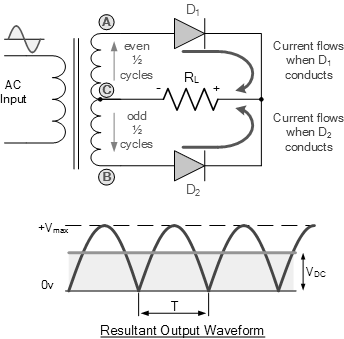A full-wave rectifier circuit consists of two power diodes connected to a load resistor (RL), each taking it in turn to supply current to the load. When point A of the transformer is positive relative to point C, diode D1 conducts in the forward direction as indicated by the arrows.

When point B is positively charged (for a negative half cycle) relative to point C, diode D2 conducts in the forward direction and current through resistor R is in the same direction in both half cycles. Since the output voltage across the resistor R is the sum of the phasors of the two combined waveforms, this type of full-wave rectifier circuit is also known as a "bi-phase" circuit. We can see this effect quite clearly if we run the circuit in a simulator with the smoothing capacitor removed.

Simulation Waveform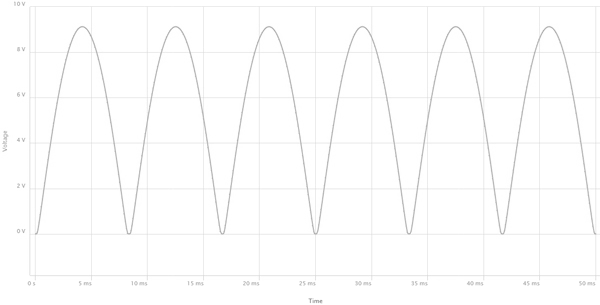As the space between each half-wave developed by each diode is now filled by the other, the average DC output voltage across the load resistor is now twice that of a single half-wave rectifier circuit and is about 0.637 Vmax of the peak voltage, assuming no losses.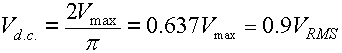Here, Vmax is the maximum peak value in one half of the secondary winding and VRMS is the RMS value as: VRMS = 0.7071Vmax. The DC current is given as: IDC = VDC/R.

The peak voltage of the output waveform is the same as before for the half-wave rectifier provided that each half-winding of the transformer has the same value of RMS voltage. To achieve a different DC voltage output, different transformer ratios can be used.

The main disadvantage of this type of full-wave rectifier circuit is the need for a larger transformer for a given output power with two separate but identical secondary windings, which makes this type of full-wave rectifier circuit expensive compared to the equivalent "full-wave bridge rectifier" circuit.

The Full Wave Bridge Rectifier

Another type of circuit that produces an output waveform similar to the full-wave rectifier circuit above is a full-wave bridge rectifier. This type of single-phase rectifier uses four individual rectifier diodes connected in a closed-loop "bridge" configuration to produce the desired output.

The main advantage of this bridge circuit is that it does not require a special centering transformer, which reduces its size and cost. The single secondary coil is connected to one side of the diode bridge network and loaded to the other side as shown below.

The Diode Bridge Rectifier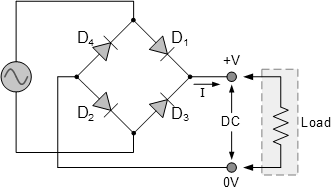The four diodes labeled D1 to D4 are arranged as "series pair" with only two diodes carrying current in each half cycle.

The Positive Half-cycle

During the positive half cycle of the power supply, diodes D1 and D2 are in series while diodes D3 and D4 are reverse biased and current flows through the load as shown below.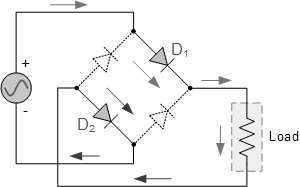The Negative Half-cycle

During the negative half cycle of the supply, diodes D3 and D4 are in series, but diodes D1 and D2 are off because they are reverse biased. The current through the load has the same direction as before.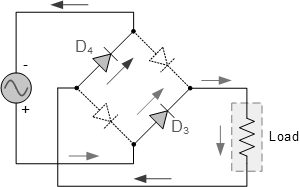Since the current through the load is DC, the voltage developed across the load is also DC as with the previous two-diode rectifier, so the average DC voltage across the load is 0.637 Vmax.

Typical Bridge Rectifier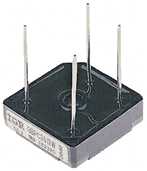In practice, however, in each half cycle current flows through two diodes instead of just one, so the magnitude of the output voltage is that the two voltages drop lower (2 * 0.7 = 1, 4 V) at the Vmax  input amplitude. The ripple frequency is now twice the supply frequency (e.g. 100Hz for a 50Hz supply or 120Hz for a 60Hz supply.)

While four individual power diodes can be used to create a full-wave bridge rectifier, pre-manufactured bridge rectifier components are available "on the shelf" in a wide range of other voltage and current sizes. each other can be soldered directly to the printed circuit board or connected by spade connectors.

A typical single-phase rectifier bridge has a cut-off angle. This cutoff indicates that the terminal closest to the corner is the positive or +ve output wire or terminal, with the opposite (diagonal) wire being the negative or output wire. The other two connection wires are for the AC input voltage of the transformer secondary winding.

Prasun Barua

Prasun Barua is an Engineer (Electrical & Electronic) and Member of the European Energy Centre (EEC). His first published book Green Planet is all about green technologies and science. His other published books are Solar PV System Design and Technology, Electricity from Renewable Energy, Tech Know Solar PV System, C Coding Practice, AI and Robotics Overview, Robotics and Artificial Intelligence, Know How Solar PV System, Know The Product, Solar PV Technology Overview, Home Appliances Overview, Tech Know Solar PV System, C Programming Practice, etc. These books are available at Google Books, Google Play, Amazon and other platforms.

*